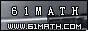Today is . WELCOME: huohai3983/44663  Home | Reg Login | All user | setHomePage | BookmarkHome | Math Test Online | Addition | Subtraction | Multiplication | Division | Time | Worksheet(JPG/PDF) | CAI | Flash Games |Recommendation articleArticle searchHome--maths--Second Grade--Subtraction using Pencil and Paper

Subtraction using Pencil and Paper

Subtracting Two Digit Numbers

How to subtract two digit numbers (for example 67 - 45).

• Place one number above the other so the tens' place digits and ones' place digits are lined up. Draw a line under the bottom number.
```67
45
```
• Subtract the two digits in the ones' place column. (7 - 5 = 2).
```67
45
2
```
• Subtract the digits in the tens' place column (6 - 4 = 2) and place the answer below the line in the tens' place column.
```67
45
22```
• If the ones' place digit that is being subtracted is larger than the top ones' place digit, decrease the top tens' place digit by one and increase the top ones' place value by ten before subtracting.

#### Find the Difference between the two Numbers.

 -     =

2006-12-16 21:25:42
Viewed 2395 times CloseGreatmathsites